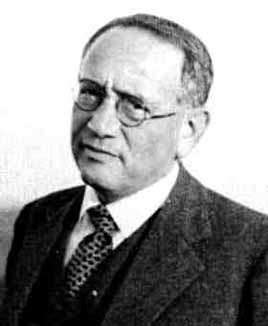George Pólya (1887 – 1985) one of the first to study rigorously random walks.

Recently a colleague of mine came with a simple question related to his second year probability classes: how to prove that an asymmetric random walk diverges to infinity almost surely, without using the Borel-Cantelli lemma, the law of large numbers, or Markov chains tools such as stopping times and recurrence/transience?

A natural answer to is prove all what is needed from scratch, which turns out to be pretty short and intuitive for the random walk. More precisely, let ${{(S_n)}_{n\geq0}}$ be an asymmetric random walk on ${\mathbb{Z}}$ started from the origin, namely ${S_0=0}$ and for any ${n\geq1}$

$S_{n}=S_{n-1}+X_{n}=X_1+\cdots+X_n,$

where ${{(X_n)}_{n\geq1}}$ is a sequence of independent and identically distributed random variables following an asymmetric Rademacher distribution:

$p:=\mathbb{P}(X_n=+1)=1-\mathbb{P}(X_n=-1)\neq\frac{1}{2}.$

Our objective is to prove that

$\mathbb{P}(\lim_{n\rightarrow\infty}S_n=-\infty)=1 \quad\text{if}\quad p<\frac{1}{2}$

and

$\mathbb{P}(\lim_{n\rightarrow\infty}S_n=+\infty)=1 \quad\text{if}\quad p>\frac{1}{2}.$

The idea is to show that ${{(S_n)}_{n\geq1}}$ is captured after a random time by a cone centered around ${\mathbb{E}(S_n)=nm}$ where ${m:=\mathbb{E}(X_i)=2p-1\neq0}$, of width ${\delta_n\ll n}$. To see it, for any ${\delta_n>0}$ to be chosen later, we introduce the event

$A_n:=\{|S_n-nm|\geq\delta_n\}.$

The Markov inequality gives (trying with ${2}$ instead of ${4}$ shows that ${2}$ is not enough)

$\mathbb{P}(A_n) =\mathbb{P}((S_n-nm)^4\geq\delta_n^4) \leq\frac{\mathbb{E}((S_n-nm)^4)}{\delta_n^4}.$

But, denoting ${X_i’:=X_i-m}$, we get ${\mathbb{E}(X’_i)=0}$ and ${S_n-nm=X_1’+\cdots+X_n’}$ and

$\mathbb{E}((S_n-nm)^4) =\mathbb{E}((X_1’+\cdots+X_n’)^4) =\mathcal{O}(n^2)\mathbb{E}(X_1’^2)+n\mathbb{E}(X_1’^4) =\mathcal{O}(n^2)$

where we used the fact that the ${X’_i}$’s are centered, independent, and bounded in ${L^4}$: ${\sup_{n\geq1}\mathbb{E}(X_n’^4)<\infty}$. Therefore for any ${\delta_n=n^\alpha}$ with ${\alpha>0}$ to be chosen later,

$\mathbb{P}(A_n)=\mathcal{O}\left(\frac{1}{n^{4\alpha-2}}\right) \quad\text{and thus}\quad \sum_{n=1}^\infty\mathbb{P}(A_n)<\infty$

as soon as ${\alpha>3/4}$. Let us introduce now the random variable

$Y:=\sum_{n=1}^\infty\mathbf{1}_{A_n}.$

The Fubini-Tonelli theorem or the monotone convergence theorem gives

$\mathbb{E}(Y) =\mathbb{E}\left(\sum_{n=1}^\infty\mathbf{1}_{A_n}\right) =\sum_{n=1}^\infty\mathbb{E}(\mathbf{1}_{A_n}) =\sum_{n=1}^\infty\mathbb{P}(A_n) <\infty.$

Since ${Y}$ takes its values in ${[0,\infty]}$, it follows that ${\mathbb{P}(Y<\infty)=1}$, in other words, almost surely, the series ${\sum_{n=1}^\infty\mathbf{1}_{A_n}}$ converges, in other words, almost surely, for ${n}$ large enough (above a random threshold), we have

$|S_n-nm|<\delta_n=n^{\alpha},$

and by taking ${3/4<\alpha<1}$, it follows that ${{(S_n)}_{n\geq1}}$ diverges to ${+\infty}$ if ${p>1/2}$ since ${m>0}$ and to ${-\infty}$ if ${p<1/2}$ since ${m<0}$ (drawing a picture with a cone may help!).

Note. This proof works beyond the case of ${\pm1}$ increments and requires only independence and boundedness in ${L^4}$. Actually the same argument gives a quick proof of the strong law of large numbers for independent random variables bounded in ${L^4}$ with same mean ${m\in\mathbb{R}}$:

$\mathbb{P}\left(\lim_{n\rightarrow\infty}\frac{S_n}{n}=m\right)=1.$

Namely since ${\mathbb{E}((\frac{S_n}{n}-m)^4)=\mathcal{O}(\frac{1}{n^2})}$, we get ${\mathbb{E}(\sum_{n=1}^\infty(\frac{S_n}{n}-m)^4)<\infty}$, and therefore ${\mathbb{P}(\lim_{n\rightarrow\infty}(\frac{S_n}{n}-m)^4=0)=1}$, which means that ${\mathbb{P}(\lim_{n\rightarrow\infty}\frac{S_n}{n}=m)=1}$. This strong law of large numbers provides in turn the almost sure divergence of the asymmetric random walk.

The argument provides also a quick proof of the first Borel-Cantelli lemma by using the Fubini-Tonelli theorem or the monotone convergence theorem. Namely, if ${{(A_n)}_{n\geq1}}$ is a sequence of events such that ${\sum_{n=1}^\infty\mathbb{P}(A_n)<\infty}$, then ${\mathbb{E}(\sum_{n=1}^\infty\mathbf{1}_{A_n})<\infty}$ and thus ${\mathbb{P}(\sum_{n=1}^\infty\mathbf{1}_{A_n}<\infty)=1}$ which means that ${\mathbb{P}(\limsup_{n\geq1}A_n)=0}$.

Note. The classical Markov chain way to prove the result consists in using the fact that ${{(S_n)}_{n\geq0}}$ is a Markov chain with state space ${\mathbb{Z}}$ and transition matrix ${\mathbf{P}_{x,y}=p\mathbf{1}_{y=x+1}+(1-p)\mathbf{1}_{y=x-1}}$. This matrix is irreducible, and therefore it suffices to show that the state ${0}$ is transient. The chain has period ${2}$ and therefore ${\mathbf{P}^{2n+1}(0,0)=0}$ for any ${n\geq0}$. For ${\mathbf{P}^{2n}_{0,0}}$, the coin tossing game due to the ${\pm1}$ increments gives ${(S_n-S_0+n)/2\sim\mathrm{Binom}(n,p)}$, which yields

$\mathbf{P}_{0,0}^{2n} =\mathbb{P}(S_{2n}=0\mid S_0=0) =\binom{2n}{n}p^n(1-p)^n \sim_{n\rightarrow\infty} \frac{\rho^n}{\sqrt{\pi n}}$

where ${\rho:=4p(1-p)}$. Now ${p\neq1/2}$ gives ${\rho<1}$, and thus

$\mathbb{E}\left(\sum_{n=1}^\infty\mathbf{1}_{S_n=0}\mid S_0=x\right) =\sum_{n=1}^\infty\mathbf{P}^{n}_{0,0} =\sum_{n=1}^\infty\mathbf{P}^{2n}_{0,0}<\infty,$

which shows that the chain is transient.

Last Updated on 2020-06-22

This site uses Akismet to reduce spam. Learn how your comment data is processed.

Syntax · Style · .